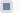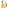Victor L. Mironov SedeonsSite menuInput formSearchStatistics Total online: 1 Guests: 1 Users: 0Welcome, Guest · RSS 26.11.2020, 06:33

# in relativistic quantum mechanics and field theory

V.L.Mironov, S.V.Mironov -  Space-time sedeons and their application in relativistic quantum mechanics and field theory
Institute for Physics of Microstructures RAS, Nizhniy Novgorod, 2014.

Available on viXra: http://vixra.org/abs/1407.0068

V.L.Mironov, S.V.Mironov - Space-time sedeons and their application in relativistic quantum mechanics and field theory

Institute for Physics of Microstructures RAS, Nizhniy Novgorod, 2014 (in Russian).

http://ipmras.ru/~Mironov/Publications/SEDEONS.pdf

Sedeonic space-time algebra

The sedeonic algebra encloses eight groups of values, which are differed with respect to spatial and time inversion.

Here indexes t and r indicate the transformations (for spatial inversion and for time inversion), which change the corresponding values. All introduced values can be integrated into one space-time object named sedeon, which is defined by the following expression:

The multiplication and commutation rules for sedeonic basis elements are presented in tables 1 and 2. In the tables the value  i  is the imaginary unit.

The publications conserning application of sedeons in field theory

V.L.Mironov, S.V.Mironov - Noncommutative sedeons and their application in field theory // ArXiv http://arxiv.org/abs/1111.4035

We present sixteen-component values "sedeons", generating associative noncommutative space-time algebra. The generalized relativistic wave equations based on sedeonic wave function and space-time operators are proposed. It is shown that the sedeonic second-order wave equation for massive field can be represented in the form of the system of Maxwell-like first-order equations for field's intensities. The sedeonic first-order Dirac-like equations for massive and massless fields are also discussed.

V.L.Mironov, S.V.Mironov, S.A.Korolev - Sedeonic theory of massless fields // ArXiv http://arxiv.org/abs/1206.5969

In present paper we develop the description of massless fields on the basis of space-time algebra of sixteen-component sedeons. The generalized sedeonic second-order equation for unified gravito-electromagnetic (GE) field describing simultaneously gravity and electromagnetism is proposed. The second-order relations for the GE field energy, momentum and Lorentz invariants are derived. We also discuss the sedeonic first-order Dirac-like equation for the massless field.

S.V.Mironov, V.L.MironovSedeonic theory of massive fields< PDF >

In the present paper we develop the description of massive fields on the basis of space-time algebra of sixteen-component sedeons. The generalized sedeonic second-order equation for the potential of baryon field is proposed. It is shown that this equation can be reformulated in the form of a system of Maxwell-like equations for the field intensities. We calculate the baryonic fields in the simple model of point baryon charge and obtain the expression for the baryon-baryon interaction energy. We also propose the generalized sedeonic first-order equation for the potential of lepton field and calculate the energies of lepton-lepton and lepton-baryon interactions.

V.L.Mironov, S.V.Mironov – Reformulation of relativistic quantum mechanics equations with non-commutative sedeons // Applied mathematics, 4(10C), 53-60 (2013).

We present sixteen-component values "sedeons", generating associative non-commutative space-time algebra. The generalized relativistic wave equations based on sedeonic wave function and space-time operators are proposed. We demonstrate that sedeonic second-order wave equation for massive field can be reformulated as the quasi-classical equation for the potentials of the field or in equivalent form as the Maxwell-like equations for the field intensities. The sedeonic first-order Dirac-like equations for massive and massless fields are also discussed.

V.L.Mironov, S.V.Mironov – Sedeonic equations of gravitoelectromagnetism // Journal of Modern Physics, 5(10), 917-927 (2014).

In present paper we develop the description of massless fields on the basis of space-time algebra of sixteen-component sedeons. The generalized sedeonic second-order equation for unified gravito-electromagnetic (GE) field describing simultaneously weak gravity and electromagnetism is proposed. The relations for the GE field energy, momentum and Lorentz invariants are derived. The special case of GE field described by first-order sedeonic wave equation is also discussed.

S.V.Mironov, V.L.Mironov – Sedeonic equations of massive fields // International Journal of Theoretical Physics, 54(1), 153-168 (2015).

In the present paper we develop the description of massive fields on the basis of space-time algebra of sixteen-component sedeons. The generalized sedeonic second-order equation for the potential of baryon field is proposed. It is shown that this equation can be reformulated in the form of a system of Maxwell-like equations for the field intensities. We calculate the baryonic fields in the simple model of point baryon charge and obtain the expression for the baryon-baryon interaction energy. We also propose the generalized sedeonic first-order equation for the potential of lepton field and calculate the energies of lepton-lepton and lepton-baryon interactions.Free web hostinguCoz